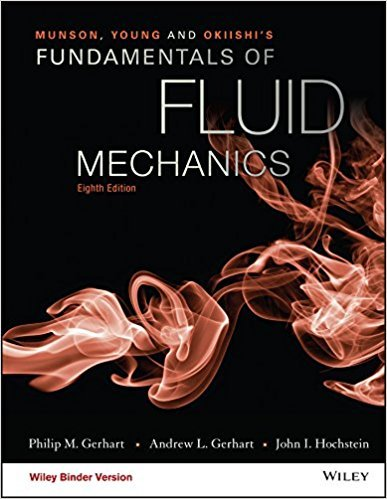×
Get Full Access to Fundamentals Of Fluid Mechanics - 8 Edition - Chapter 11.8 - Problem 11.63
Get Full Access to Fundamentals Of Fluid Mechanics - 8 Edition - Chapter 11.8 - Problem 11.63

×

# Air enters a 4-cm-square galvanized steel duct with p0 =150 kPa, T0 = 400K, and V1 = 120ISBN: 9781119080701 456

## Solution for problem 11.63 Chapter 11.8

Fundamentals of Fluid Mechanics | 8th Edition

• Textbook Solutions
• 2901 Step-by-step solutions solved by professors and subject experts
• Get 24/7 help from StudySoup virtual teaching assistantsFundamentals of Fluid Mechanics | 8th Edition

4 5 1 338 Reviews
23
3
Problem 11.63

Air enters a 4-cm-square galvanized steel duct with p0 =150 kPa, T0 = 400K, and V1 = 120 m/s. (Note: = 2.2 105N s/m2).(a) Compute the maximum possible duct length for these conditions.(b) If the actual duct length is 0.75 times the maximum value,calculate the mass flow rate, the exit pressure, and the stagnationpressure.(c) If the actual duct length is 1.3 times the maximum value forthe stated conditions, compute the new mass flow rate and inletvelocity. Assume a low back pressure and use the same value of fas used in the maximum possible duct length case.

Step-by-Step Solution:
Step 1 of 3

Chapter 3: Transport Layer 3.1 Intro and Transport-Layer Services  A transport-layer protocol provides for logical communication between application processes running on different hosts  Transport protocol run on end systems o THey break application layer messages into segments and pass those onto the network layer, then on the way back they receive these segments and assemble them into messages for the app layer  TCP and UDP are transport-layer protocols

Step 2 of 3

Step 3 of 3

##### ISBN: 9781119080701

This full solution covers the following key subjects: . This expansive textbook survival guide covers 112 chapters, and 1357 solutions. Since the solution to 11.63 from 11.8 chapter was answered, more than 262 students have viewed the full step-by-step answer. The answer to “Air enters a 4-cm-square galvanized steel duct with p0 =150 kPa, T0 = 400K, and V1 = 120 m/s. (Note: = 2.2 105N s/m2).(a) Compute the maximum possible duct length for these conditions.(b) If the actual duct length is 0.75 times the maximum value,calculate the mass flow rate, the exit pressure, and the stagnationpressure.(c) If the actual duct length is 1.3 times the maximum value forthe stated conditions, compute the new mass flow rate and inletvelocity. Assume a low back pressure and use the same value of fas used in the maximum possible duct length case.” is broken down into a number of easy to follow steps, and 96 words. The full step-by-step solution to problem: 11.63 from chapter: 11.8 was answered by , our top Science solution expert on 03/16/18, 03:21PM. Fundamentals of Fluid Mechanics was written by and is associated to the ISBN: 9781119080701. This textbook survival guide was created for the textbook: Fundamentals of Fluid Mechanics, edition: 8.

## Discover and learn what students are asking

Calculus: Early Transcendental Functions : Space Coordinates and Vectors in Space
?In Exercises 1-4, plot the points in the same three-dimensional coordinate system. (a) (3, -2, 5)

Calculus: Early Transcendental Functions : Second-Order Nonhomogeneous Linear Equations
?Verifying a Solution In Exercises 1-4,verify the solution of the differential equation. Solution D

Chemistry: The Central Science : Basic Concepts of Chemical Bonding
?Write the Lewis symbol for atoms of each of the following elements: (a) Al, (b) Br, (c) Ar, (d) Sr.

Unlock Textbook Solution